E X A M P L E S O F M O D E L
S T R U C T U R E S
damien lejay
April 2017
Abstract. We give an introduion to the andard model ruures on the
category of unbounded chain complexes by deﬁning ﬁr model ruures on
the category of Abelian groups.
contents
1 Reminder on why we like model categories 1
2 The father of all examples 2
3 Model ruures on Abelian groups 4
3.1 The andard projeive model ruure 5
3.2 The andard injeive model ruure 7
4 The model ruure on chain complexes over a ﬁeld 9
5 Model ruures on chain complexes of Abelian groups 12
5.1 The andard projeive model ruure 12
5.2 The andard injeive model ruure 13
References 14
1 reminder on why we like model categories
The derived category
D(Z)
is by deﬁnition the localisation of the category of
chain complexes where the set of maps we would like to invert is the set of all
quasi-isomorphisms Q:
D(Z) Q
1
Ch(Z)
In fa, it is deﬁned as the model of localisation whose set of objes is the
set of all chain complexes (the localisation of a category is only deﬁned up to
equivalences of categories).
Given a category
C
and a set
S
of arrows of
C
, if
C
is known to be a
U
-small
category, then any model of the localisation
S
1
C
is garanted to be equivalent
to a
U
-small category. Apart from that, essentially nothing is know on the
concrete description of the localisation. If we choose the model which has
the same objes, then the diculty is to underand the set of arrows. In
full generality, the be we know is that it can be described as some huge
‘zig-zags’.
The situation is much better in the following two cases.
The set S admits a calculus of fraions;
The category C can be enhanced into a model category.
In the ﬁr case, the morphisms
X Y
of the localisation may be described
by ‘fraions’ of one of the two types:
X
s
Z
f
Y || X
f
Z
s
Y
These notes were written for the ‘derived seminar’ I organised at IBS Centre for geometry and
physics in Pohang. Copyright (C) Damien Lejay 2017.
1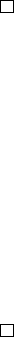with
s S
, depending on whether
S
admits a right or left calculus of frac-
tions .
In the second case, a new model for the localisation may be proposed. The
ruure of model category allows us to isolate the subcategory of ﬁbrant-
coﬁbrant objes. The localisation called the homotopy category in this
context — is then given by deﬁning maps between ﬁbrant-coﬁbrant objes as
homotopy equivalence classes of morphisms in the category C.
The ﬁr case is less convenient to work with because before arting to
compute the equivalence classes of arrows up to homotopy, one ﬁr has to
replace objes by equivalent ﬁbrant-coﬁbrant objes. Alas, the set
Q
of all
quasi-isomorphisms does not have a calculus of fraions.
Proposition. The set
Q
of quasi-isomorphisms in
Ch(Z)
calculus of fraions.
Proof. We will show that
Q
does not satisfy the — left or right — cancellab-
ility condition.
Let
A
be the chain complex
Z
Id
Z
and let
f : A Z
be the projeion
on the ﬁr term. Then
s : 0 A
is a quasi-isomorphism such that
f s = 0
but it is impossible to ﬁnd a quasi-isomorphism
s
: Z B
such that
s
f = 0
(since f is surjeive, that would mean s
= 0).
In the other direion let
i : Z A
be the injeion to the second term.
Then if
t
is the quasi-isomorphism
A 0
, we have
t i = 0
. But since
i
is
injeive, it is impossible to have a quasi-ismorphism
t
: B Z
such that
i t
= 0.
Hence, we are doomed to ﬁnd a model ruure on the category of chain
complexes. The minimum requirement to be able to ﬁnd such ruure with
weak equivalences the set
Q
is that
Q
be able under retras and satisfy the
2 out of 3 property.
Proposition. The set
Q
of quasi-isomrophisms of
Ch(Z)
is able under retras
and satisﬁes the 2 out of 3 property.
Proof. The 2 out of 3 property is an immediate consequence of the funtori-
ality of the homology funor C 7→ H
(C).
The same is true for the ability under retras, if
g
is a retra of a
given chain map
f
then,
H
(g)
is a retra of
H
(f )
and any retra of an
isomorphism is an isomorphism.
Remark. More generally, given any funor
F : C D
, the set of arrows
S
deﬁned by
s S
if and only if
F(s)
is an isomorphism, will satisfy the 2 out of
3 property and be able under retras.
This means aually that the two condition imposed on a set to be weak
equivalences of a model ruure are faily weak: for any category
C
and set
of arrows
S
, the set of arrows
W
in
C
that become and isomorphism in the
localisation
S
1
C
is able under retra and satisﬁes the 2 out of 3 property.
And of course we have S
1
C W
1
C.
2 the father of all examples
Before inveigating the possible model ruures on the category of chain
complexes, we shall ﬁr focus on more elementary Abelian categories.
Let
F
Vect
F
denote the category of veor
spaces over
F
. We shall classify all the possible model ruures on
Vect
F
and we shall discover that only two sets of arrows can be taken as set of weak
equivalences for a model ruure.
The key to classify model ruures on
Vect
F
is to ﬁr classify lifting
syems (L, R). We shall see that there are only a handful of them.
2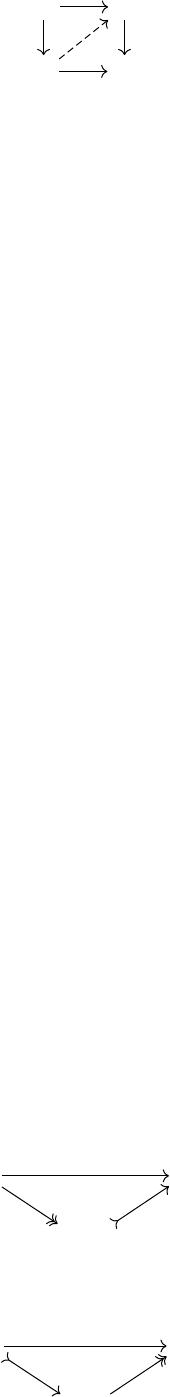So let
(L, R)
be a lifting syem i.e the elements of
L
are the maps
l
such
that the following diagram can be ﬁlled up
A C
B D
l
r
for every arrow
r R
. In the same fashion, the set
R
is required to be deﬁned
as the maps
r
that lift again all maps of
L
. Hence by deﬁnition,
L
contains
all isomorphisms.
Case 1. L = {iso}. In this case, R = {all} and we have a ﬁr pair
(iso, all)
Case 2.
L
contains a map
f
that not injeive. Any map
f
retras to the
zero map
Ker f 0
; in this case, since the kernel of
f
is not zero,
f
retras
to the zero map
π : F 0.
So by the ability properties of lifting syems, we know that
π
is in
L
.
Computing the set of maps that can be lifted again π, we get
π
l
= {injections}
Let ι be the zero injeion
ι : 0 F
By computation we have
l
ι = {surjections}.
Hence, we get that
{surjections} =
l
ι
l
(f
l
) L
Case 3.
L
contains a map
f
that is not surjeive. Then in this case, it is
possible to retra f to ι. By computation,
ι
l
= {surjections}
Since we also have
l
π = {injections},
we have
{injections} =
l
π
l
(f
l
) L
Conclusion. Since
L
is also able by composition, we have only four possible
choices for L and they correspond to the following lifting pairs
(iso, all) (inj, surj) (surj, inj) (all, iso)
Notice that all those four lifting pairs are also weak faorisation syems.
Indeed, the faorisation for the pair (surj, inj) is the image faorisation
A B
Im(f )
f
and it is the graph faorisation for the pair (inj, surj):
A B
A B
f
Γ
π
B
3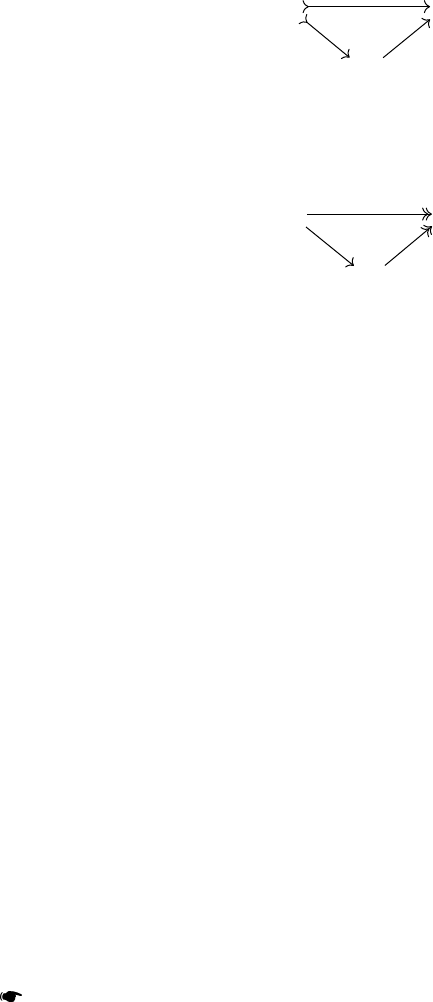Getting back to ﬁnding all model ruures, we are looking for three sets
of maps
(W, C, F)
such that
(W C, F)
and
(C, W F)
are weak faoriion
syems and
W
satisﬁes the 2 out of 3 property. Since there are only four
lifting syems, we can deduce that any set
W
has to contain either
iso, inj, surj
or be the set of all arrows.
Suppose
W
contains the set of all injeive maps. Then give any map
f : A B, since we have the following commutative diagram
0 B
A
f
by the 2 out of 3 property, f is in W and W = {all}.
Suppose now that
W
contains the set of all surjeive maps. Then for any
f : A B, the following diagram
A 0
B
f
and the 2 out of 3 property means that f is in W and W = {all}.
We can know li all the model ruures on the category Vect
F
:
(iso, all, all), it is the trivial model ruure;
(all, iso, all)
, it is also a trivial model ruure but the homotopy cat-
egory in this case is the point;
(all, all, iso), analogous to the previous one;
(all, surj, inj);
(all, inj, surj).
Remark. The same inveigations can be made for
Set
the category of sets.
However, since it is usually impossible to retra to the empty set, there
are a total of 9 dierent model ruures on
Set
with 3 dierent homotopy
categories.
As we can see, only the la two model ruures are not trivial. But the
very la one is far more useful than the other. We shall call it the andard
model ruure on
Vect
F
. The reason behing this is that both the set of ﬁbrant
objes and coﬁbrant objes are reduced to
0
for the model ruure given
by the triplet
(all, surj, inj)
. Hence, there is no space for tweaking: if we
would like to change some parts of the ruure and keep the ﬁbrations for
examples, then whatever we do, the set of ﬁbrant-coﬁbrant objes is ill
going to be reduced to a point. The same happen if we would like to keep the
coﬁbrations. On the opposite, in the andard model ruure, every obje is
both ﬁbrant and coﬁbrant!
Theorem (Experimental fa). All useful model categories are based on the
andard model ruure.
3 model structures on abelian groups
The ﬁr main dierence between
Ab
the category of Abelian groups and
Vect
F
is that we ill do not have a complete classiﬁcation of objes in
Ab
[
2
,
3
].
As a consequence, it is impossible to compute all the model ruures on
Ab
. What we shall do inead, is to build model ruures that mimic the
andard model ruure on Vect
F
.
Fa. — The pair (inj, surj) is not a lifting pair in Ab.
4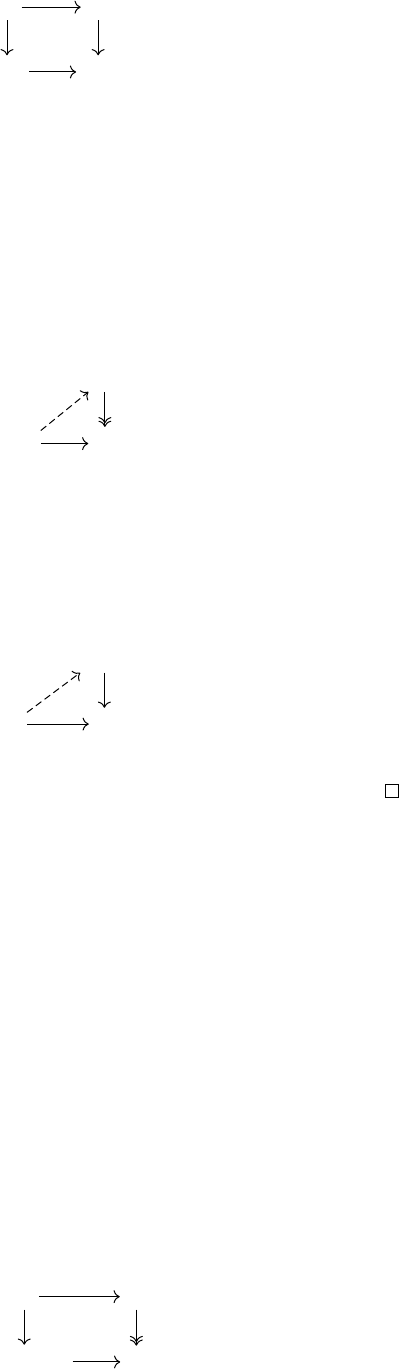To see this, have a look to the following commutative square,
0 Z
Z
2
Z
2
mod
2
Id
3.1 The andard projeive model ruure
Idea 1. — Keep the ﬁbration and look for the corresponding left part of the
pair.
Fir let us look at the corresponding coﬁbrant objes.
Definition 3.1. An abelian group
P
is called projeive if the map
0 P
satisﬁes the left lifting property again surjeions. That is, we can always ﬁnd a
lift for the following types of diagrams,
X
P Y
Proposition 3.2. The projeive Abelian groups are the free Abelian groups.
Proof. If
P
is a free Abelian group then it is projeive as any map with
domain P is only determined by the image of elements of a basis of P.
Suppose P is projeive and let L(P) be the free Abelian group generated
by the elements of
P
. Then because
P
is projeive we get the following
retraion
L(P)
P P
Id
So
P
can be seen as a subgroup of a free Abelian group and since
Z
is a PID,
it means P itself is a free Abelian group.
We can now generalise this setting to describe all the maps that can be
lifted again surjeions.
Proposition. A map
f : A B
is inside
l
surj
if and only if it is a (split)
injeion with a free cokernel.
Proof. If
f
is an injeion with a free cokernel, then it has to be split (thanks
to the lifting property of free modules), so we can write
B A Coker f
and
f
is the identity of
A
. Hence, we can split the problem into a lifting
problem for
Coker f
and
Id
A
. We have already deﬁned the former to be
objes that solve that very problem and isomorphisms can always be lifted.
Suppose now that
f
satisﬁes the left lifting property. Denote by
π
the
projeion
π : B Coker f
To show that the cokernel is free, we will show that it is projeive. Let us be
given any diagram
0 X
Cocker f Y
p
g
5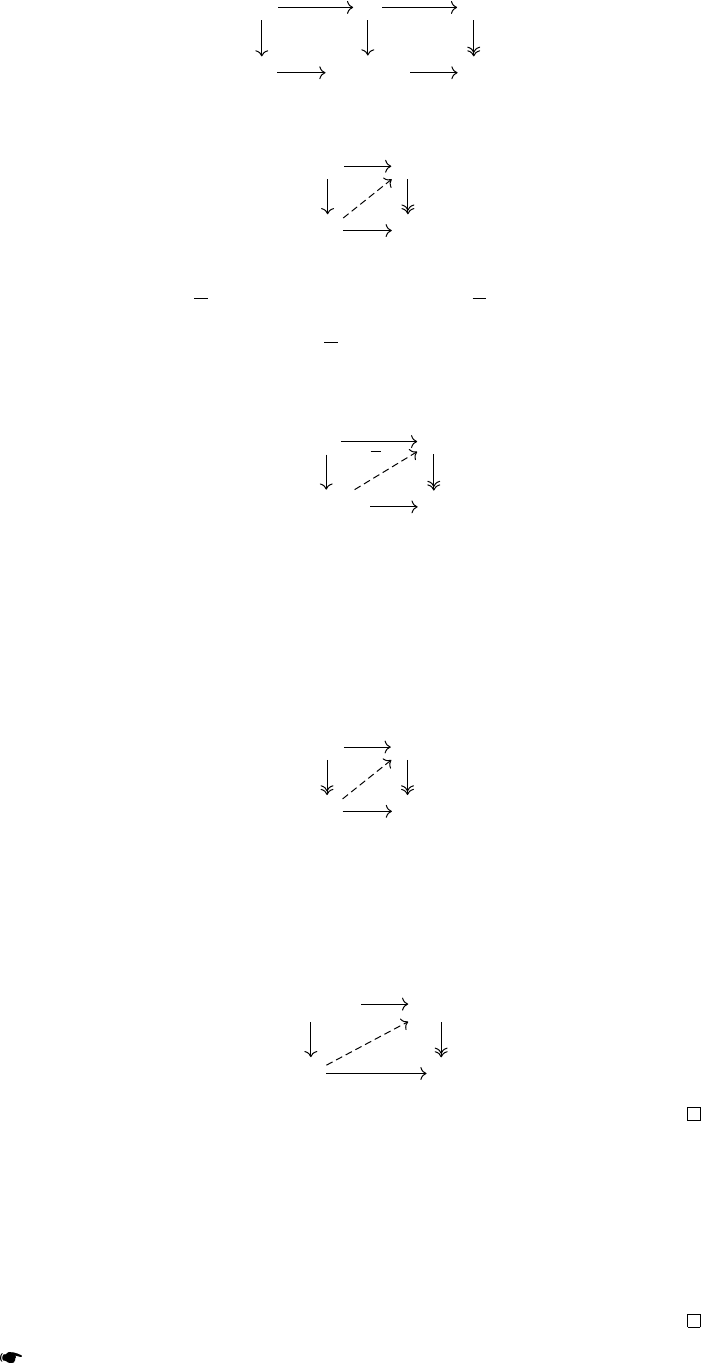and we wish to lift
g
. Composing,
g
with
π
gives us a map
B Y
whose
composition with
f
is equal to zero. Hence we can complete the former
diagram into
A 0 X
B Cokerf Y
f
p
π
g
Using the lifting property of f , we can complete the square in
A X
B Y
0
f
p
gπ
ϕ
But since
ϕ
composed with
f
is zero, by the universal property of the cokernel,
we get a new map ϕ : Coker f X such that ϕ = ϕ π. So we now have
p ϕ π = g π
and since π is an epimorphism we get the following lifting
0 X
Coker f Y
p
g
ϕ
This proves that the cokernel has to be projeive. Since maps with a projeive
cokernel split, we can then rewrite B as the sum
B Im f Coker f
And we can now reri our attention to the case where the cokernel is zero.
In this case the following diagram
A A
B B
Id
f f
Id
tells us that we can split A into
A Ker f B
This la diagram will tell us that the kernel of f has to be zero:
Ker f B Ker f
B 0
π
Ker f
π
B
Corollary. The pair made of injeive maps with free cokernels on one side
and surjeive maps on the other side, is a lifting pair.
Proof. All we need to prove is that any surjeion has the right lifting
property again any injeive map
f : A B
with free cokernel. But as we
have already said, such a map can be split into the sum of an isomorphism
(which can be lifted) and the zero map
0 Coker f
which by deﬁnition of
projeiveness can also be lifted.
Theorem. There exis a model ruure (W, C, F) on Ab where:
W is the set of all maps;
6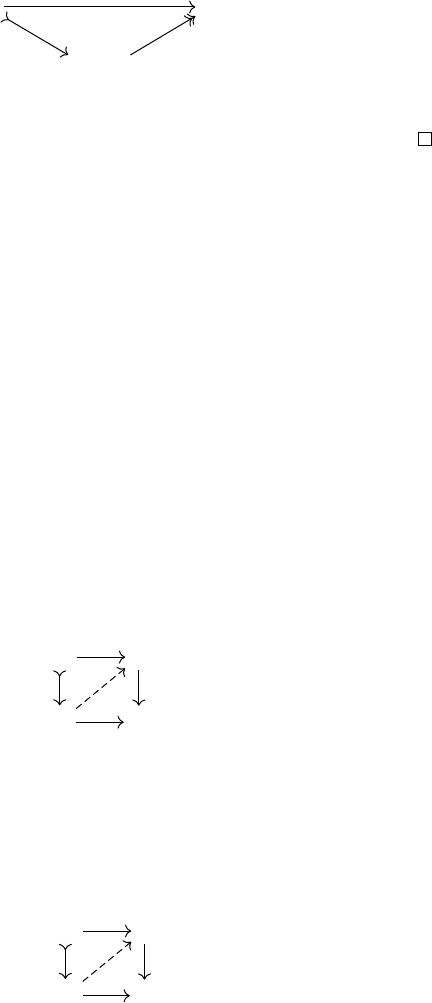F is the set of all surjeive maps;
C is the set of all injeive maps with free cokernel.
This model category is called the andard projeive model ruure.
Proof. Since
W F = F
and
W C = C
, the only thing that is left to
prove is the faoriion property. Let
f : A B
be any map between Abelian
groups. Let
L(B)
be the free Abelian group with basis the elements of
B
,
the canonical map
π : L(B) B
is surjeive. We obtain the sought for
faorisation as
A B
A L(B)
f
ι
A
f +π
where
ι
A
is the inclusion on the ﬁr faor. The cokernel of
ι
A
is
L(B)
and it
is free.
Remark. The andard projeive model ruure will exis for every
category of modules over a commutative ring. Ju replace free Abelian
groups by the corresponding projeive modules over the given ring (if the
ring is not a PID).
Remark. In this model ruure every obje is ﬁbrant but the only coﬁbrant
objes are the free Abelian groups. Many times in the proves, we have used
the fa that for every Abelian group
B
, there exis a surjeion
L(B) B
with
L(B)
a free Abelian group. This is nothing else than the coﬁbrant replacement
of B.
3.2 The andard injeive model ruure
Idea 2. — Keep the weak equivalences and the coﬁbrations.
In this case let us give a name to the new ﬁbrant objes.
Definition 3.3. An Abelian group
I
, is injeive if it satisﬁes the right lifting
property again all injeions, i.e if every such diagrams can be ﬁlled,
X I
Y 0
Proposition. The injeive Abelian groups are the divisible Abelian groups,
that is the Abelian groups
I
such that for every
a I
and
n Z
, there exis
b I
such that a = nb.
Proof. The divisibility condition for an Abelian group
I
is equivalent to
being able to ﬁll the following diagrams
Z I
Z 0
×n
Hence every injeive Abelian group is divisible. Conversely, given a divisible
Abelian group
I
, a map
f : X I
and an inclusion
X Y
, we will show
that
f
can be extended to
Y
. Let
y
be an element of
Y
that is not in
X
. Then
either
y
is free relative to
X
and
f
may be extended to
X Zy
by choosing
any element r I and letting f (x + ny) = f (x) + n f (y) be the extension.
Either there exis an element
x X
and a prime number
p Z
such that
py = x
7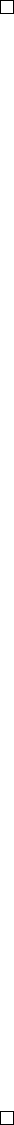in which case, thanks to the divisibility of
I
, there is an element
r I
such
that
pr = f (x)
. Then to any element
x + my X + Zy
, the extended map
is given by
f (x + my) = f (x) + m r
. The extended map is correly deﬁned
since f (py) = pr = f (x).
This extension can be performed on any submodule of
Y
on which
f
has previously been extended. The results then comes from (transﬁnite)
induion.
Definition 3.4. For a prime number
p
, let
Z
p
be the Abelian group colimit of
the diagram of inclusions Z
p
n
, Z
p
n+1
.
Remark. Since every cyclic group Z
p
n
can be represented as the quotient
Z
p
n
Z 1/p
n
/Z
the group Z
p
may be seen as
Z
p
Z[1/p]/Z.
where
.
denotes group generation and
[.]
denotes ring generation. It can also
be described as
Z
p
Q/pZ.
Proposition (Classiﬁcation of injeive Abelian groups). Every injeive
Abelian group is a sum of the groups Q and Z
p
for every prime p.
Proof. Fir
Q
and
Z
p
are injeive because they are divisible. This is
obvious for the ﬁr one and a consequence of Bezout theorem for the second
one.
Let
I
be any injeive Abelian group, we will show that
I
splits as the sum
of its injeive subgroups ‘divisibly generated’ by one element. Let
x
be an
element of
I
. If
x
is not a torsion element, since
I
is divisible, it deﬁnes an
injeion
ι
x
: Q I
and as
Q
is injeive,
I
splits into a sum
I Q J
where
J
is also divisible (every quotient of a divisible group is divisible).
Hence we can now suppose that
I
is equal to its torsion subgroup. For
a given prime number
p
, let
x
be an element of
p
-torsion of
I
. Since
I
is
divisible, for every k 1, there exis an element x
k
I such that
x = p
k1
x
k
The subgroup of
I
generated by the
x
k
for
k 1
is isomorphic to
Z
p
. The
map sends the generators
p
k
to
x
k
and all we need to show is that every
linear equation
N
X
k=1
a
k
x
k
= 0
with all
a
k
integer numbers not divisible by
p
, image of a element
P
N
k=1
a
k
/p
k
in
Z
p
, is in fa impossible. For every
k
, by conruion
p
k
x
k
= 0
. It follows
that
p
N1
a
N
x
N
= 0
. But since
p
N
x
N
= 0
it means that
p
divides
a
N
which is
impossible unless a
N
= 0. This shows that the built map
Z
p
I
is injeive. The end of the proof consis in a usual (transﬁnite) induion.
Remark. By the classiﬁcation of divisible Abelian groups, we see that they
are exaly the Abelian groups where the ruure of
(Z, +)
-module can be
extended to a ruure of (Q, +)-module.
Proposition (Fibrant replacement). The induion funor
Q
(Z,+)
: Ab Mod
(Q,+)
8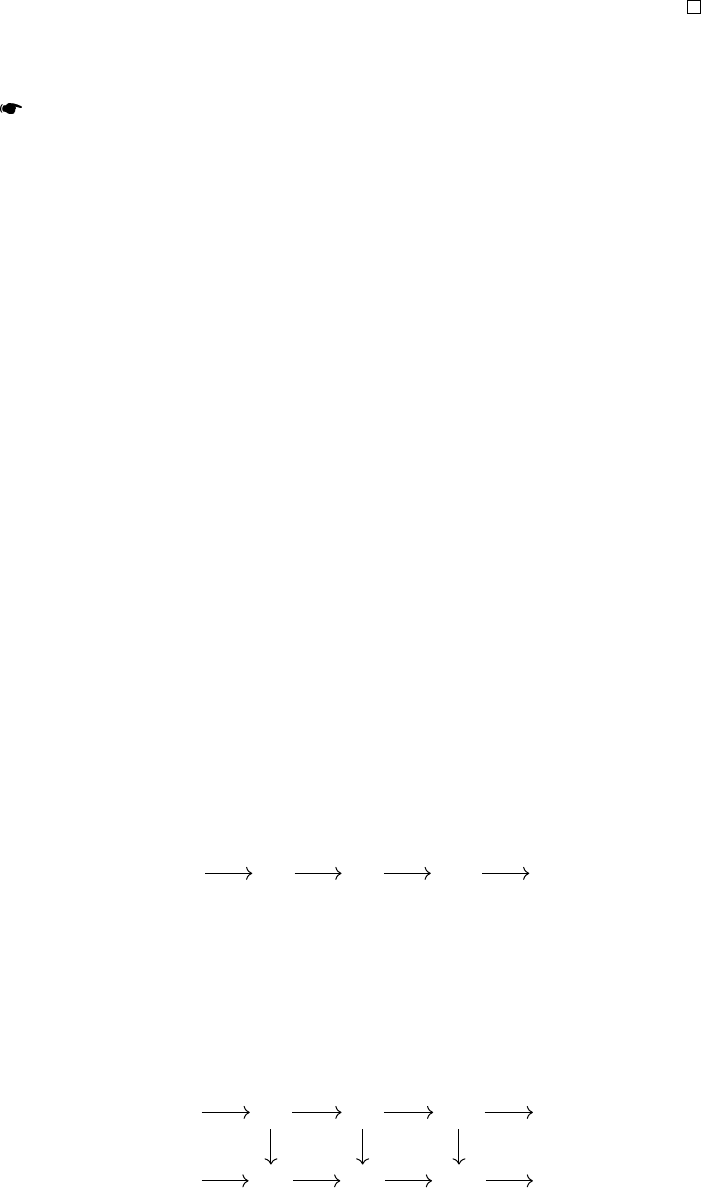is left adjoint to the forgetful funor and is such that the unit of the adjunion is
a monomorphism i.e for every Abelian group A, the canonical map
A , Q
(Z,+)
A
is an injeion.
In particular, we get the following replacements:
Z , Q ; Z
p
n
, Q/p
n
Z Z
p
Proof. The adjunion is a andard result in the theory of modules over
monoids. The only thing we have to prove is that the unit is a monomorphism.
Let
a
be any element of
A
, then its image by the unit is
(0, a)
which is equal
to 0 if and only if a is equal to zero in A.
Now that we have dealt with the necessary prerequisites, we can describe
the new model ruure.
Theorem. There exis a model ruure on the category of Abelian groups
where
any map is a weak equivalence;
a map is a coﬁbration if and only if it is injeive;
a map is a ﬁbration if and only if it is a (split) surjeion with divisible
kernel.
This model ruure is called the andard injeive model ruure.
The proof of this theorem is a complete mirror of the proof of the exience
of the projeive model ruure (once you know how to build a ﬁbrant
replacement). Indeed, it is the projeive model ruure on the category
Ab
op
.
Remark. As we see, the key fa about the category of Abelian groups is
that we are always able to ﬁnd, for every group
A
, a surjeive map to
A
with
free domain and an injeive map from
A
to a divisible group. We say that
the category of Abelian groups has both enough projeives and injeives.
4 the model structure on chain complexes over a field
Let us move on to the category
Ch(F)
of unbounded chain complexes over
F
. This time we will build a model ruure with non-
trivial weak equivalences, namely the set Q of quasi-isomorphisms.
But ﬁr we may warn the reader of the following fa.
Proposition. Any chain complex in Ch(F) retras to its homology.
Proof. Let
... C
1
C
0
C
1
...
2
1
0
1
be any chain complex over
F
. Let us denote by
B
n
the image of the map
n+1
in
C
n
and let
Z
n
be the kernel of
n
inside
C
n
. Finally let
H
n
be the quotient
Z
n
/B
n
. Since on a ﬁeld, every submodule is a dire summand, we get that
for every n,
C
n
B
n
H
n
B
n1
.
Hence it is very raighforward to build a retraion map
... C
1
C
0
C
1
...
... H
1
H
0
H
1
...
2
1
π
1
0
π
0
1
π
1
0 0 0 0
9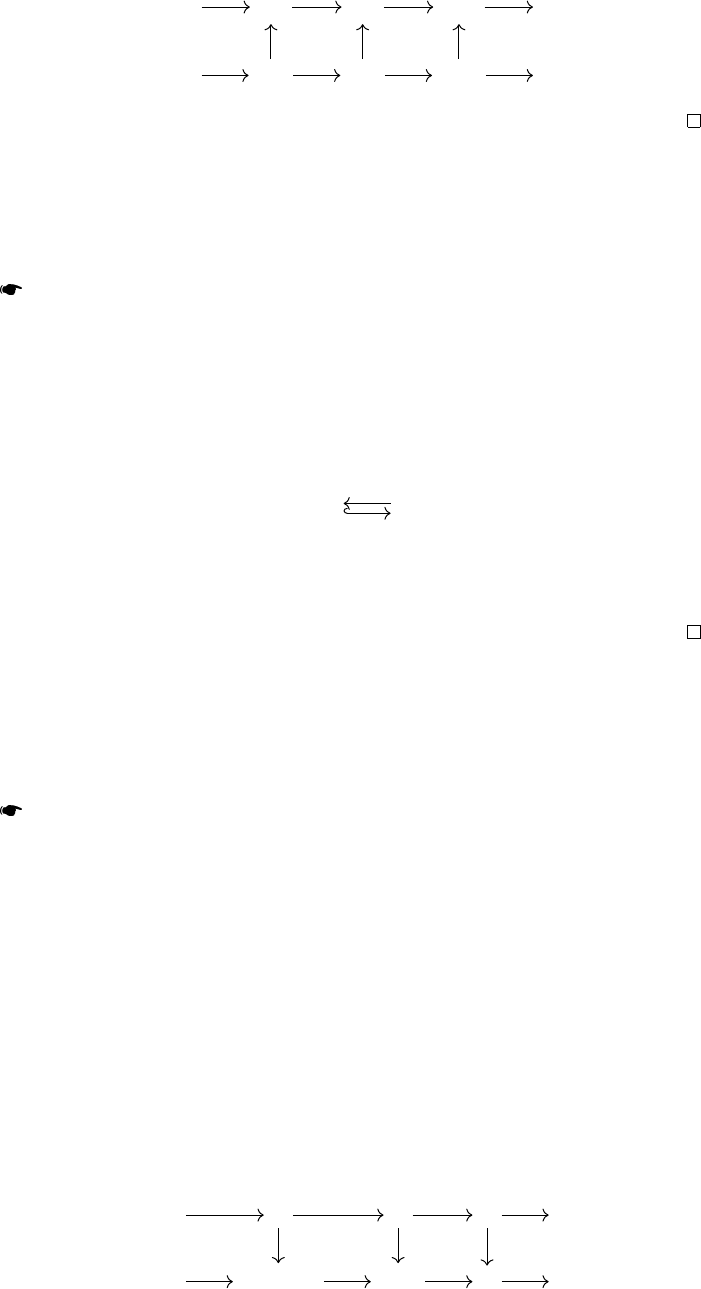with raighforward seion
... C
1
C
0
C
1
...
... H
1
H
0
H
1
...
2
1
0
1
0 0
ι
1
0
ι
0
0
ι
1
Remark. Although every chain complex retras to its homology, it is not
true that every map of chain complexes f retras to H(f ).
As a consequence, we have the following description of the derived cat-
egory of chain complexes over F.
Theorem. The homotopy category
D(F)
is equivalent to the category of graded
veor spaces Mod
Z
F
.
Proof. Thanks to the previous proposition, the homology funor
H : Ch(F) Vect
Z
F
is left adjoint to the inclusion of graded veor spaces inside chain complexes
Vect
Z
F
Ch(F)
H
with π being the unit of the adjunion and ι being the counit.
Moreover, a chain map is an isomorphism precisely if its image by
H
is
an equivalence of graded veor spaces. Thus, the derived category is even a
reﬂeive localisation of Ch(F).
As a consequence, we do not need any model ruure on the category of
chain complexes to be able to underand its homotopy category. Nonethe-
less, underanding the andard model ruure on the category of chain
complexes over a ﬁeld will be an important ep toward the model ruures
we are going to build on the category of chain complexes of Abelian groups.
Theorem. There exis a model ruure on the category of chain complexes
over F where
the weak equivalences are the quasi-isomorphism;
the ﬁbrations are the epimorphism;
the coﬁbrations are the monomorphisms.
This model rure is called the andard model ruure.
Proof. In view of the total symmetry of the given ruure, we shall
only prove that the pair made of trivial coﬁbrations and ﬁbrations is a weak
faorisation syem. The same proof applied to
Ch(F)
op
will prove that
coﬁbrations and trivial ﬁbrations also form a weak faorisation syem.
Let us ﬁr setup some notations. We will call
ι
n
the following trivial
coﬁbration
... 0 0 0 ...
... F[n + 1] F[n] 0 ...
Id
F
A map of complexes
f
has the right lifting property again
ι
n
if and only if
f
n+1
is surjeive, hence
{ι
n
}
l
nZ
= {ﬁbrations}
10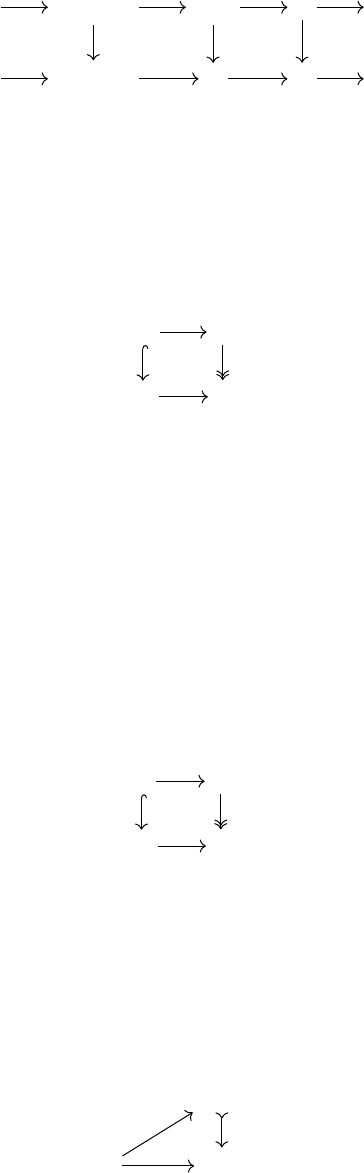Let
π
n
denote the surjeion
π
n
: F[n] 0
. A map
f
has the left lifting
property again
π
n
if and only if
H
n
(f )
is injeive. Finally let us denote by
p
n
the following ﬁbration
... F[n + 1] F[n] 0 ...
... F[n + 1] 0 0 ...
Id
F
Id
F
A map of chain complexes
f
has the left lifting property again
p
n
if and
only if H
n+1
(f ) is surjeive and f
n
is injeive. As a consequence
l
{π
n
, p
n
}
nZ
= {trivial coﬁbrations}
Laly we need to show that it is aually possible to lift any of our deﬁned
trivial coﬁbrations again a ﬁbration. Let
f : X Y
be a trivial coﬁbration
and g : R S be a ﬁbration and let be given a commutative square
X R
Y S
r
f
g
s
We will conru a map
ϕ : Y R
to ﬁll up this diagram. A andard rategy
would be to art lifting
ϕ
0
and then induively build the other
ϕ
n
but since
we are dealing with unbounded chain complexes there isn’t really a right
place to art and we will see that being able to lift is in fa some kind of a
global property of a complex. Let us adopt another rategy.
Fir we may split Y as a sum
Y X Coker f
and since
f
is a quasi-equivalence, its cokernel is aually quasi-isomorphic to
zero. As it is always possible to lift an isomorphism, we can suppose that
X
is
zero and that
Y
is quasi-isomorphic to zero. Hence what we shall prove is that
trivial coﬁbrant objes lift againts ﬁbrations. We wish to lift the following
diagram
0 R
Y S
g
t
Since
g
n
is a surjeion for every
n
, it is possible to ﬁnd a map
ϕ
n
: Y
n
R
n
lifting s
n
. This gives us a map ϕ of graded veor spaces but it may fail to be
a chain map. Let ψ be deﬁned as the failure of ϕ to be a chain map:
ψ
n
=
n+1
ϕ
n+1
ϕ
n
n+1
By conruion
ψ
is a chain map from
Y
to
R
and since
ϕ
is a lift again
g
,
the image of ψ is contained in (Ker g),
(Ker g)
Y R
g
ψ
Now thanks to the exaness of the complex
Y
, it is aually homotopic to zero
(since exa complexes are split over a ﬁeld). Hence, we can ﬁnd a homotopy
h between ψ and 0:
ψ
n
=
n+1
h
n+1
h
n
n+1
We now deﬁne a new map
τ = ϕ h
11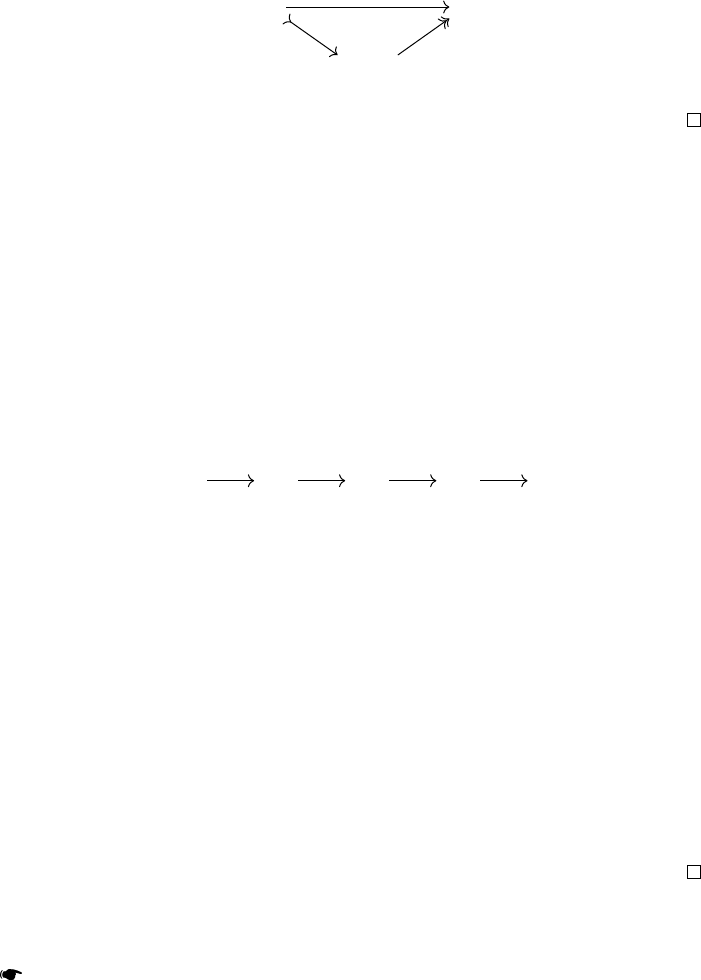By conruion
τ
is a chain map from
Y
to
R
. By conruion also, since
h
has its values in Ker g, we have
g τ = t
And the diagram is ﬁlled.
We now approch the very ﬁnal ep: showing that any map can be faored
into a trivial coﬁbration followed by a ﬁbration. Let
f : X Y
be any map of
chain complexes. Let also
e
Y
be the chain complex quasi-isomorphic to zero
such that
e
Y
0
= Y
,
e
Y
1
= Y
and the only non-zero map is the identity of
Y
. Let
π :
e
Y Y
be the ﬁbration deﬁned by projeion on the
0
faor. Then we can
faor the map f as follows,
X Y
X
e
Y
f
ι
f π
where ι is the inclusion on the ﬁr faor.
5 model structures on chain complexes of abelian groups
5.1 The andard projeive model ruure
The situation for the andard projeive model ruure on chain complexes
of modules over a ring is aually a bit worse than for the projeive model
ruure on the category of modules or on chain complexes over a ﬁeld.
Indeed, it is not true for example that for any ring, a chain complex of free
modules is going to have the left lifting property again all epimorphisms.
Here is a famous counter example: the ground ring is
Z
4
and the following
chain complex is only made of free modules,
. . . Z
4
Z
4
Z
4
. . .
×2 ×2 ×2 ×2
It is an exa complex but as it does not split, it is not homotopic to zero! Hence
it can’t be a coﬁbrant obje and as a consequence, ﬁnding free resolutions is
no longer enough to build coﬁbrant replacement... On a PID, the situation is
aually much better.
Proposition. Any exa chain complex of free Abelian groups is homotopic to
zero.
Proof. Let
C
be an exa chain complex of free Abelian groups. For every
n Z
,
B
n
C
n
is a subgroup of a free Abelian groups, hence it is also free.
Moreover, since
C
is exa, the quotient
C
n
/B
n
is isomorphic to the image
B
n1
which is free (as a subgroup of a free Abelian group) so that
C
n
can
aually be split
C
n
B
n
B
n1
And split exa chain complexes are homotopic to zero.
Thanks to this proposition we can now deﬁne the andard model ruure
on the category of chain complexes of Abelian groups.
Theorem. There exis a model ruure on the category
Ch(Z)
of chain
complexes of Abelian groups where:
the weak equivalences are the quasi-isomorphisms;
the ﬁbrations are the epimorphisms;
the coﬁbrations are the split monomorphisms with cokernel made of free
Abelian groups.
12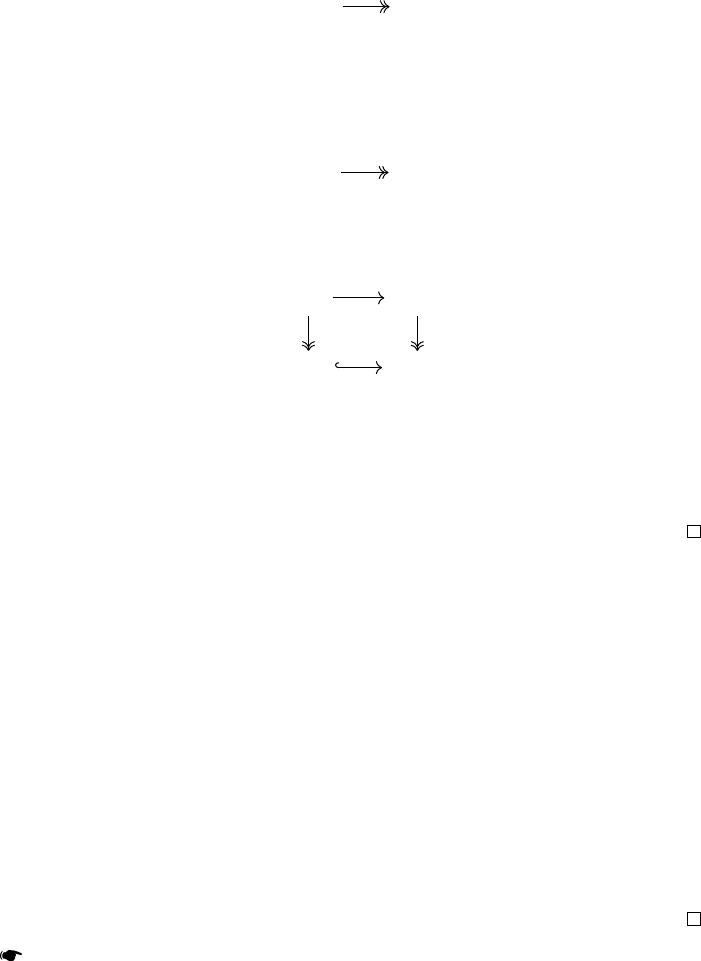This model ruure is called the andard projeive model ruure.
Remark. In the case of the category of chain complexes over a non PID ring,
the andard model ruure ill exisits but the coﬁbrant objes are a bit
dierent: there are the complexes
P
made of projeive modules such that
every map
P K
with
K
quasi-isomorphic to zero, is homotopic to the zero map. We shall call
such a chain complex dg-projeive.
The proof of the exience of the projeive model ruure is essentially
the same as the one on chain complexes over a ﬁeld; the previous proposition
guaranties that chain complexes of free Abelian group are coﬁbrant.
In order to prove the two faorisations some extra work is needed and in
particular, the following propoion mu be used.
Proposition. Given a chain complex of Abelian groups
C
there exis a chain
complex of free Abelian groups L and a trivial ﬁbration
L C
Proof. We are going to give the reciepe to build a dg-projeive resolution
(the fa that the ring is a PID is not relevant here). Fir truncate on the right
the chain complex and then build induively a projeive resolution. This is
always possible since there are enough projeives. We then have
P
0
C
0
Then funorially complete the projeive resolution into a projeive res-
olution
P
n
C
n
for
n 0
. I.e for every negative
n
we want to have the
following diagram
P
n
P
n1
C
n
C
n1
Then take the ﬁltered colimit indexed by
ω
0
to get a dg-projeive resolu-
tion. This happens because both weak equivalences and ﬁbrations are able
under ﬁltered colimits. As such, any ﬁltered colimit of dg-projeive chain
complexes is again dg-projeive and every ﬁltered colimit of resolutions is a
resolution. Laly, every right bounded chain complex of projeive modules is
dg-projeive
5.2 The andard injeive model ruure
For injeive modules, the same problems appear as with the projeive mod-
ules. Namely, some chain complexes of injeive modules may not be ﬁbrant
in the andard model ruure. As before, the situation on a PID is much
better.
Proposition. Any exa chain complex of divisible Abelian groups is homotopic
to zero.
Proof. The proof boils down to the following fa: every quotient of a
divisible group is again divisible. This means that given any complex of
divisible groups
C
, for every
n
the boundaries subgroup
B
n
C
n
is divisible
and then
C
n
may be split into
C
n
B
n
B
n1
if
C
were exa. This means
that C is homotopic to zero.
Theorem. There exis a model ruure on the category of chain complexes of
Abelian groups where:
the weak equivalences are the quasi-isomorphisms;
13
the coﬁbrations are the monomorphisms;
the ﬁbrations are the epimorphisms with kernel made of divisible Abelian
groups.
This model ruure is called the andard injeive model ruure.
In the same way that we reﬁne the deﬁnition of coﬁbrant objes, we may
deﬁne a dg-injeive chain complex as a complex
I
such that for every map
K I
with K quasi-isomorphic to zero, is homotopy equivalent to zero.
But contrarily to the projeive case, we need to use one more assumption
to be able to build a dg-injeive resolution for every chain complex: we need
to use the fa that ﬁltered colimits in the Abelian category we are working
in are exa i.e that they preserve the monomorphisms. Apart from that the
proves are totally similar.
references
 Gabriel P. and Zisman M., Calculus of fraions and homotopy theory,
vol. 35. Springer Berlin Heidelberg, 1967.
 Fuchs L., Inﬁnite Abelian groups. Vol. I. Academic Press, 1970.
 Fuchs L., Inﬁnite Abelian groups. Vol. II. Academic Press, 1973.
14